# CS229编程8：异常检测与推荐系统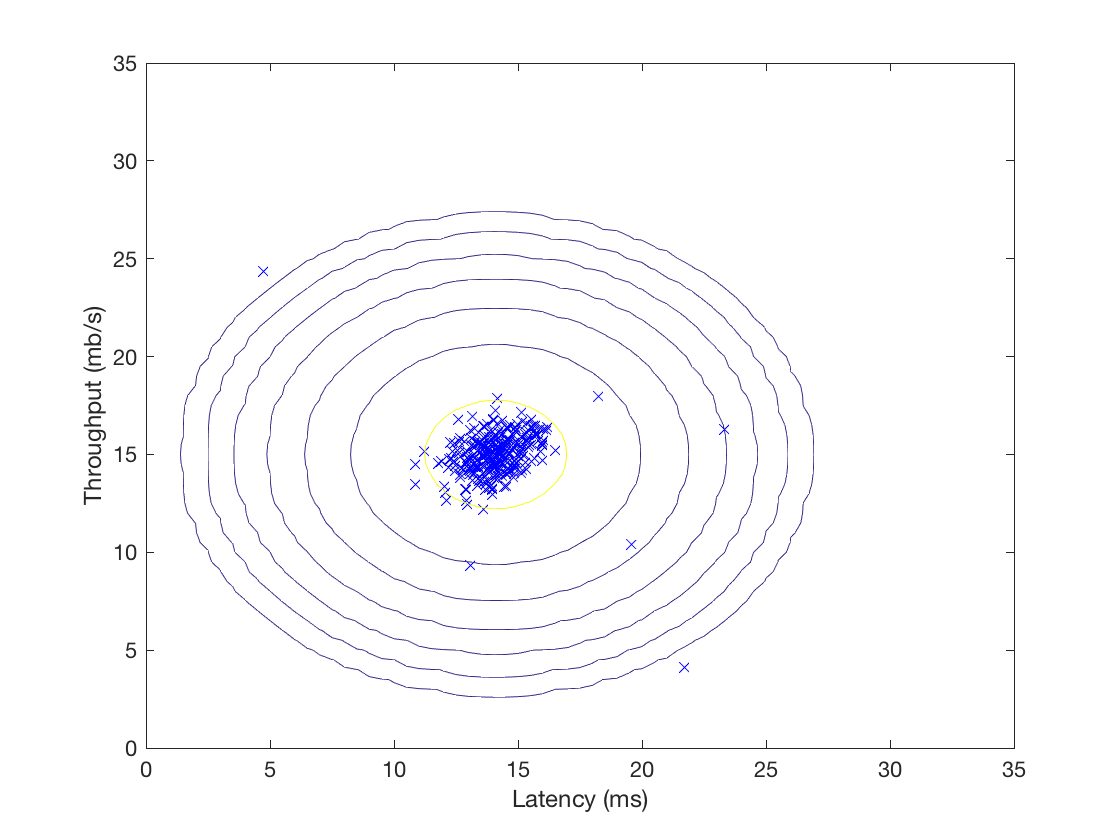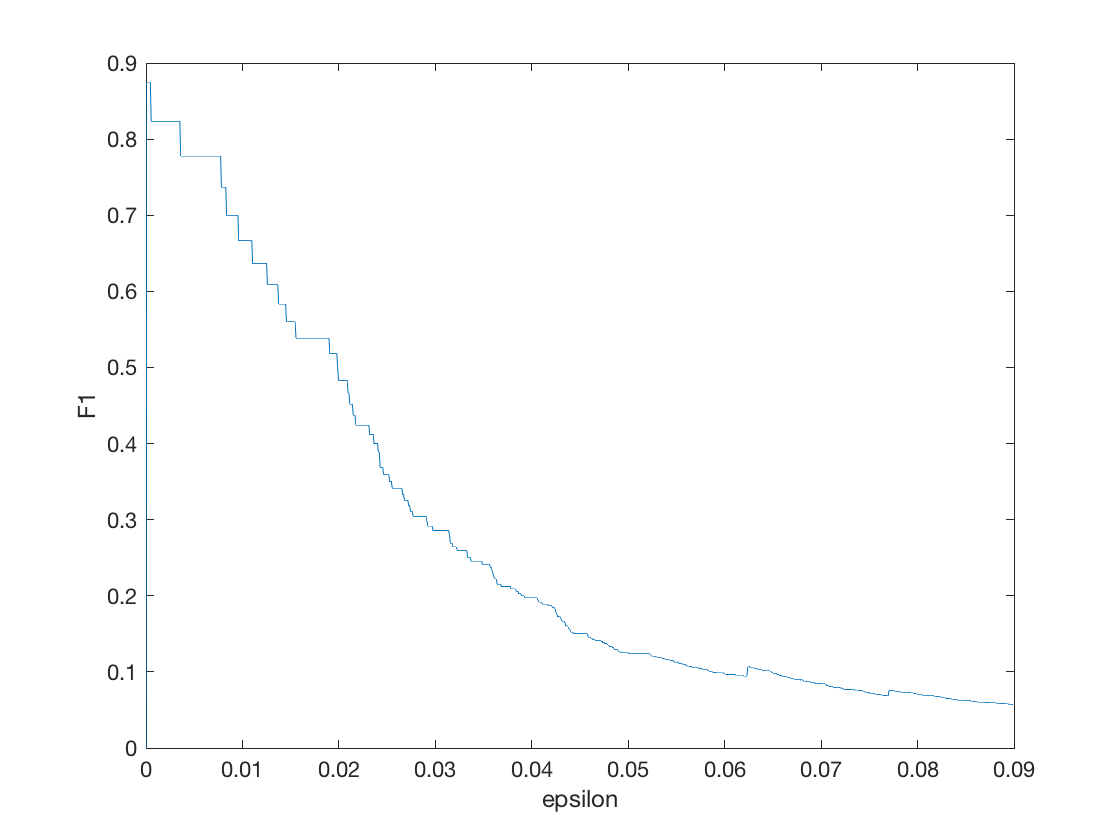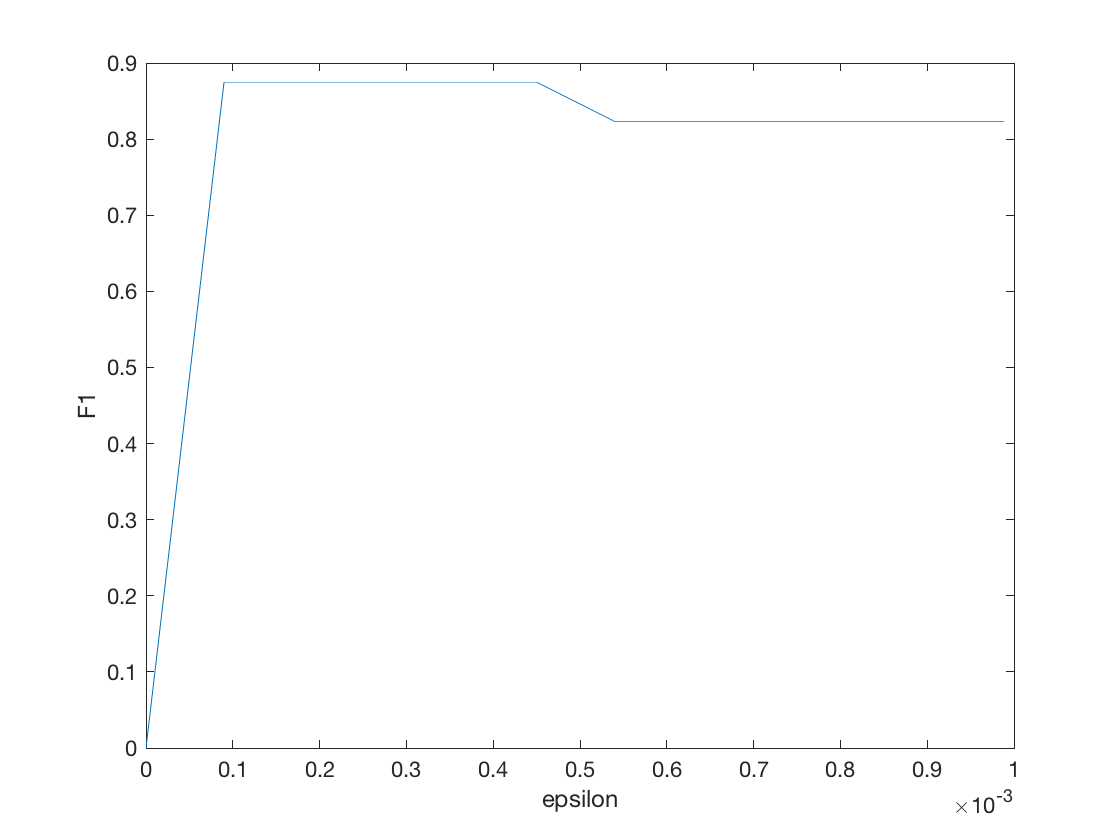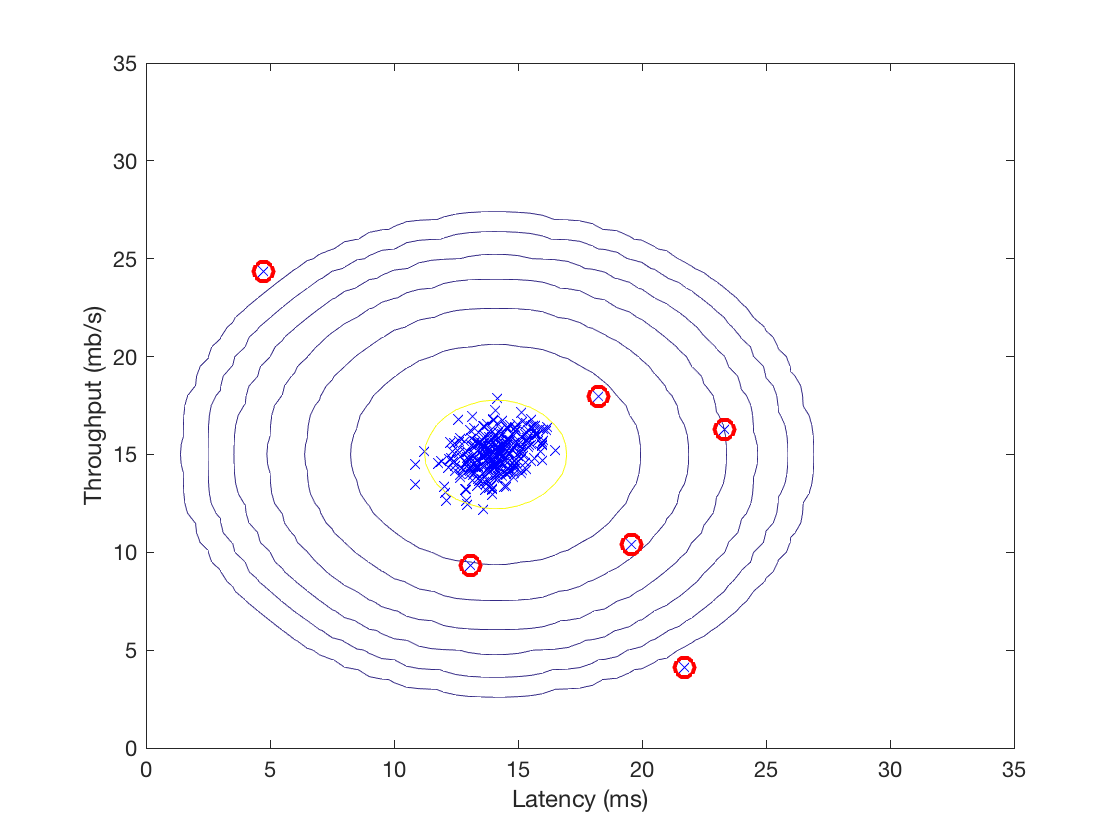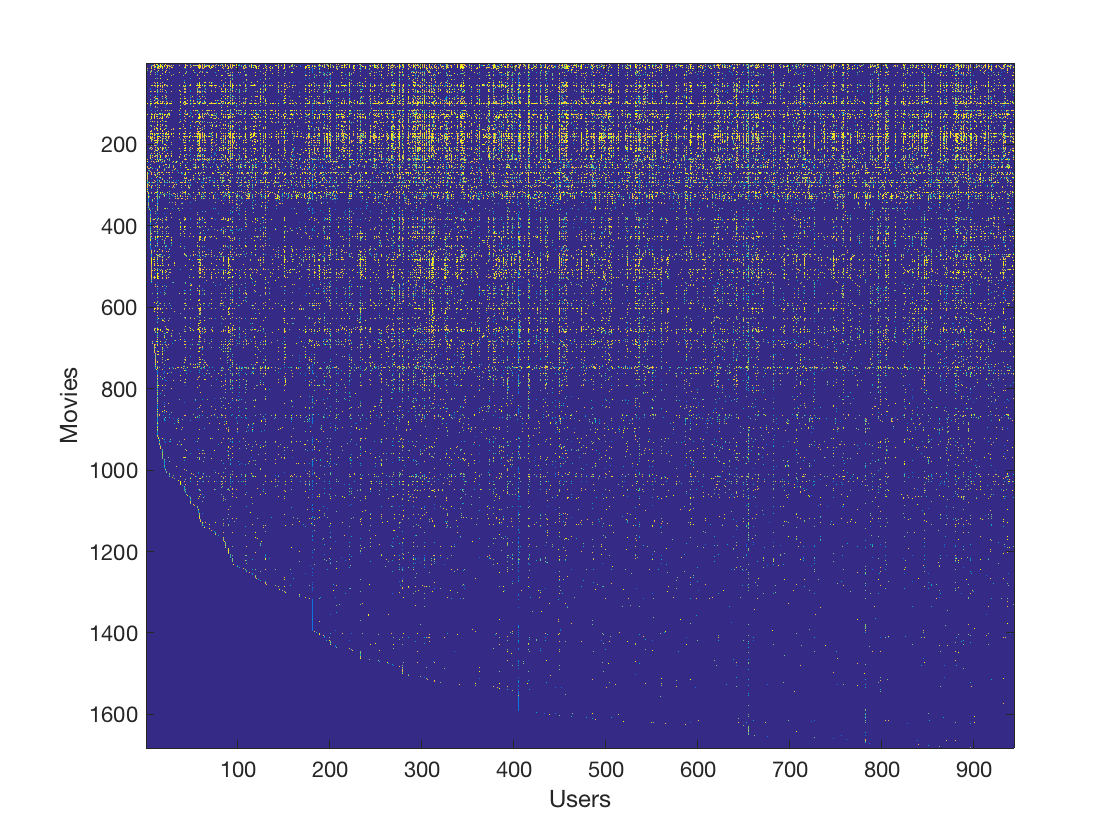斯坦福ML（Matlab）公开课最后一次编程练习，实现了异常检测算法并应用于服务器异常检测，然后利用协同过滤实现一个电影推荐系统。至此又填完一个坑，matlab感觉也入门了## 异常检测

%% ================== Part 1: Load Example Dataset  ===================
%  We start this exercise by using a small dataset that is easy to
%  visualize.
%
%  Our example case consists of 2 network server statistics across
%  several machines: the latency and throughput of each machine.
%  This exercise will help us find possibly faulty (or very fast) machines.
%

fprintf('Visualizing example dataset for outlier detection.\n\n');

%  The following command loads the dataset. You should now have the
%  variables X, Xval, yval in your environment

%  Visualize the example dataset
plot(X(:, 1), X(:, 2), 'bx');
axis([0 30 0 30]);
xlabel('Latency (ms)');
ylabel('Throughput (mb/s)');

fprintf('Program paused. Press enter to continue.\n');
pause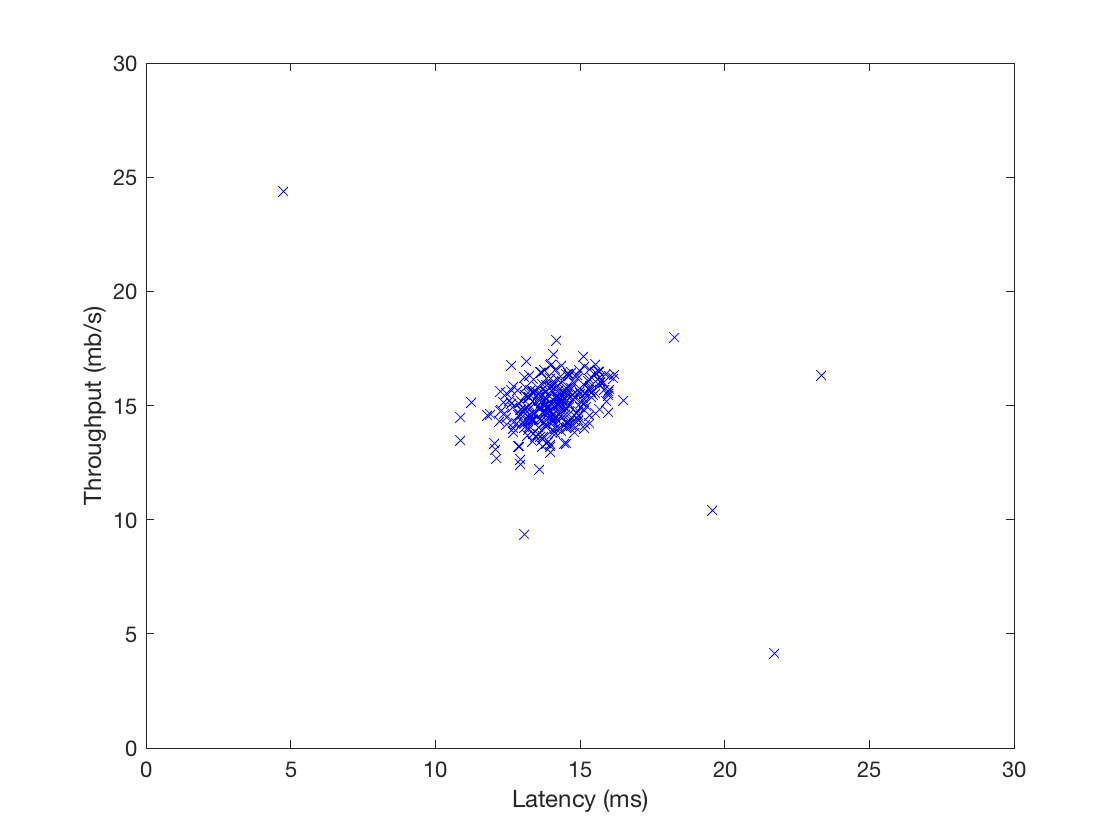### 高斯分布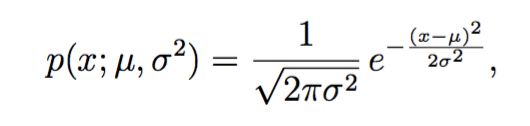function p = multivariateGaussian(X, mu, Sigma2)
%MULTIVARIATEGAUSSIAN Computes the probability density function of the
%multivariate gaussian distribution.
%    p = MULTIVARIATEGAUSSIAN(X, mu, Sigma2) Computes the probability
%    density function of the examples X under the multivariate gaussian
%    distribution with parameters mu and Sigma2. If Sigma2 is a matrix, it is
%    treated as the covariance matrix. If Sigma2 is a vector, it is treated
%    as the \sigma^2 values of the variances in each dimension (a diagonal
%    covariance matrix)
%

k = length(mu);

if (size(Sigma2, 2) == 1) || (size(Sigma2, 1) == 1)
Sigma2 = diag(Sigma2);
end

X = bsxfun(@minus, X, mu(:)');
p = (2 * pi) ^ (- k / 2) * det(Sigma2) ^ (-0.5) * ...
exp(-0.5 * sum(bsxfun(@times, X * pinv(Sigma2), X), 2));

end

### 估计高斯分布的参数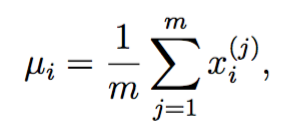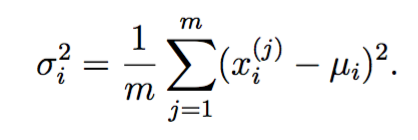function [mu sigma2] = estimateGaussian(X)
%ESTIMATEGAUSSIAN This function estimates the parameters of a
%Gaussian distribution using the data in X
%   [mu sigma2] = estimateGaussian(X),
%   The input X is the dataset with each n-dimensional data point in one row
%   The output is an n-dimensional vector mu, the mean of the data set
%   and the variances sigma^2, an n x 1 vector
%

% Useful variables
[m, n] = size(X);

% You should return these values correctly
mu = zeros(n, 1);
sigma2 = zeros(n, 1);

% ====================== YOUR CODE HERE ======================
% Instructions: Compute the mean of the data and the variances
%               In particular, mu(i) should contain the mean of
%               the data for the i-th feature and sigma2(i)
%               should contain variance of the i-th feature.
%
mu = mean(X)';
%var normalizes V by N-1 if N>1,where N is the sample size.
sigma2 = var(X) * (n -1) / n;

for i = 1:n
X(:,i) = X(:,i) - mu(i);
end
sigma2 = 1 / m * sum(X .^2)';

% =============================================================

end

%% ================== Part 2: Estimate the dataset statistics ===================
%  For this exercise, we assume a Gaussian distribution for the dataset.
%
%  We first estimate the parameters of our assumed Gaussian distribution,
%  then compute the probabilities for each of the points and then visualize
%  both the overall distribution and where each of the points falls in
%  terms of that distribution.
%
fprintf('Visualizing Gaussian fit.\n\n');

%  Estimate my and sigma2
[mu sigma2] = estimateGaussian(X);

%  Returns the density of the multivariate normal at each data point (row)
%  of X
p = multivariateGaussian(X, mu, sigma2);

### 可视化高斯分布

function visualizeFit(X, mu, sigma2)
%VISUALIZEFIT Visualize the dataset and its estimated distribution.
%   VISUALIZEFIT(X, p, mu, sigma2) This visualization shows you the
%   probability density function of the Gaussian distribution. Each example
%   has a location (x1, x2) that depends on its feature values.
%

[X1,X2] = meshgrid(0:.5:35);
Z = multivariateGaussian([X1(:) X2(:)],mu,sigma2);
Z = reshape(Z,size(X1));

plot(X(:, 1), X(:, 2),'bx');
hold on;
% Do not plot if there are infinities
if (sum(isinf(Z)) == 0)
contour(X1, X2, Z, 10.^(-20:3:0)');
end
hold off;

end

meshgrid接受一个向量，创建两个大小相等的方阵，一个矩阵的每行都是向量的拷贝，另一个每列都是向量的拷贝。这样在一个矩阵中任取一个元素作为x，在另一个矩阵的对应位置取到y，就能得到指定区间的格子坐标。### 选择阈值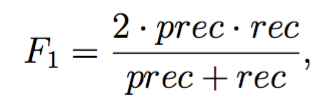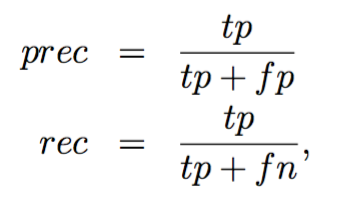tp代表真阳，fp代表假阳，fn表示假阴。

function [bestEpsilon bestF1] = selectThreshold(yval, pval)
%SELECTTHRESHOLD Find the best threshold (epsilon) to use for selecting
%outliers
%   [bestEpsilon bestF1] = SELECTTHRESHOLD(yval, pval) finds the best
%   threshold to use for selecting outliers based on the results from a
%   validation set (pval) and the ground truth (yval).
%

bestEpsilon = 0;
bestF1 = 0;
F1 = 0;

stepsize = (max(pval) - min(pval)) / 1000;
for epsilon = min(pval):stepsize:max(pval)

% ====================== YOUR CODE HERE ======================
% Instructions: Compute the F1 score of choosing epsilon as the
%               threshold and place the value in F1. The code at the
%               end of the loop will compare the F1 score for this
%               choice of epsilon and set it to be the best epsilon if
%               it is better than the current choice of epsilon.
%
% Note: You can use predictions = (pval < epsilon) to get a binary vector
%       of 0's and 1's of the outlier predictions
predictions = (pval < epsilon);
tp = sum((predictions == 1) & (yval == 1));
fp = sum((predictions == 1) & (yval == 0));
fn = sum((predictions == 0) & (yval == 1));
if tp + fp ~= 0
prec = tp / (tp + fp);
else
prec = 0;
end
if tp + fn ~= 0
rec = tp / (tp + fn);
else
rec = 0;
end
if prec + rec ~= 0
F1 = 2 * prec * rec / (prec + rec);
else
F1 = 0;
end

% =============================================================

if F1 > bestF1
bestF1 = F1;
bestEpsilon = epsilon;
end
end

end

%% ================== Part 4: Multidimensional Outliers ===================
%  We will now use the code from the previous part and apply it to a
%  harder problem in which more features describe each datapoint and only
%  some features indicate whether a point is an outlier.
%

%  Loads the second dataset. You should now have the
%  variables X, Xval, yval in your environment

%  Apply the same steps to the larger dataset
[mu sigma2] = estimateGaussian(X);

%  Training set
p = multivariateGaussian(X, mu, sigma2);

%  Cross-validation set
pval = multivariateGaussian(Xval, mu, sigma2);

%  Find the best threshold
[epsilon F1] = selectThreshold(yval, pval);

fprintf('Best epsilon found using cross-validation: %e\n', epsilon);
fprintf('Best F1 on Cross Validation Set:  %f\n', F1);
fprintf('# Outliers found: %d\n', sum(p < epsilon));
fprintf('   (you should see a value epsilon of about 1.38e-18)\n\n');
pause

Best epsilon found using cross-validation: 8.990853e-05
Best F1 on Cross Validation Set:  0.875000
(you should see a value epsilon of about 8.99e-05)F1的确有一个先增后减的过程。## 推荐系统

### 电影数据集

%% =============== Part 1: Loading movie ratings dataset ================
%  You will start by loading the movie ratings dataset to understand the
%  structure of the data.
%

%  Y is a 1682x943 matrix, containing ratings (1-5) of 1682 movies on
%  943 users
%
%  R is a 1682x943 matrix, where R(i,j) = 1 if and only if user j gave a
%  rating to movie i

%  From the matrix, we can compute statistics like average rating.
fprintf('Average rating for movie 1 (Toy Story): %f / 5\n\n', ...
mean(Y(1, R(1, :))));

R矩阵是布尔矩阵，可直接作为索引用。

%  We can "visualize" the ratings matrix by plotting it with imagesc
imagesc(Y);
ylabel('Movies');
xlabel('Users');

fprintf('\nProgram paused. Press enter to continue.\n');
pause;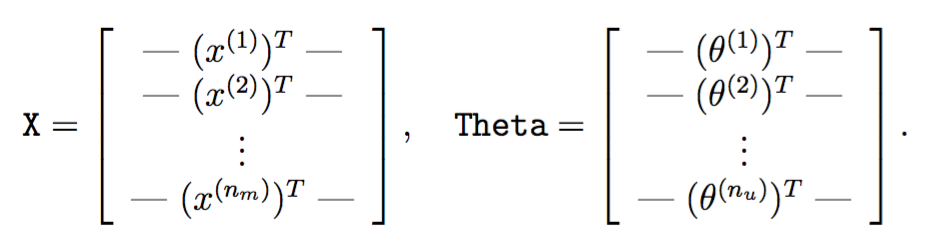X大小为电影数*特征数，第i行代表第i部电影的特征，Theta大小为用户数*特征数，第j行代表第j个用户对应的参数。在本次试验中，特征数为100。

### 协同过滤学习算法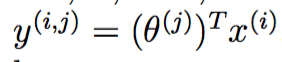### 损失函数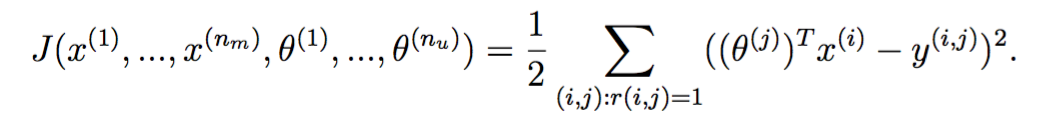### 协同过滤梯度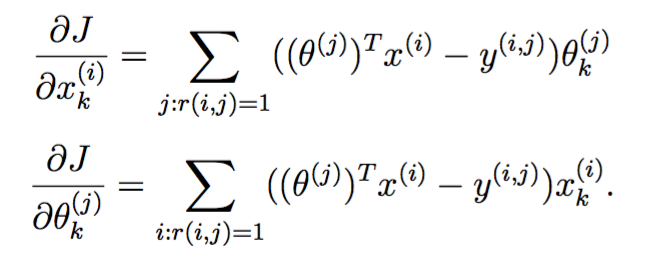### 正则化的损失函数和梯度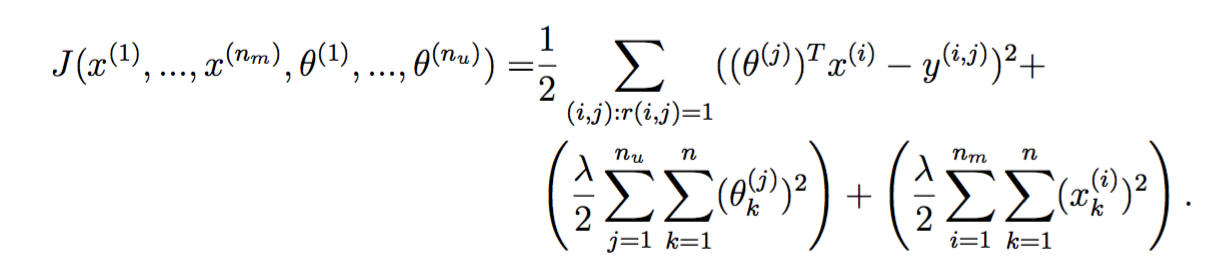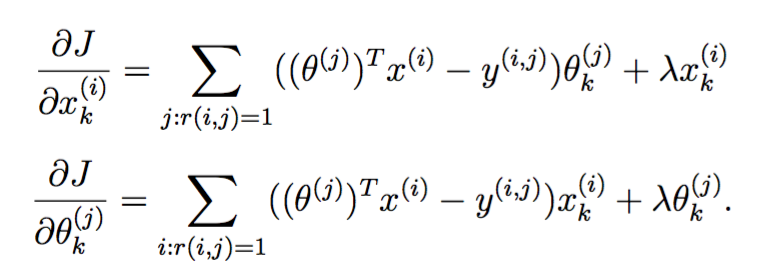function [J, grad] = cofiCostFunc(params, Y, R, num_users, num_movies, ...
num_features, lambda)
%COFICOSTFUNC Collaborative filtering cost function
%   [J, grad] = COFICOSTFUNC(params, Y, R, num_users, num_movies, ...
%   num_features, lambda) returns the cost and gradient for the
%   collaborative filtering problem.
%

% Unfold the U and W matrices from params
X = reshape(params(1:num_movies*num_features), num_movies, num_features);
Theta = reshape(params(num_movies*num_features+1:end), ...
num_users, num_features);

% You need to return the following values correctly
J = 0;

% ====================== YOUR CODE HERE ======================
% Instructions: Compute the cost function and gradient for collaborative
%               filtering. Concretely, you should first implement the cost
%               function (without regularization) and make sure it is
%               matches our costs. After that, you should implement the
%               gradient and use the checkCostFunction routine to check
%               that the gradient is correct. Finally, you should implement
%               regularization.
%
% Notes: X - num_movies  x num_features matrix of movie features
%        Theta - num_users  x num_features matrix of user features
%        Y - num_movies x num_users matrix of user ratings of movies
%        R - num_movies x num_users matrix, where R(i, j) = 1 if the
%            i-th movie was rated by the j-th user
%
% You should set the following variables correctly:
%
%        X_grad - num_movies x num_features matrix, containing the
%                 partial derivatives w.r.t. to each element of X
%        Theta_grad - num_users x num_features matrix, containing the
%                     partial derivatives w.r.t. to each element of Theta
%
M = (X * Theta' - Y) .^ 2;
J = sum(sum(R .* M)) / 2;
J = J + lambda / 2 * (sum(sum(X .^ 2)) + sum(sum(Theta .^ 2)));

X_grad = (R .* (X * Theta' - Y)) * Theta;
Theta_grad = (R .* (X * Theta' - Y))' * X;

% =============================================================

end

%% ========= Part 4: Collaborative Filtering Cost Regularization ========
%  Now, you should implement regularization for the cost function for
%  collaborative filtering. You can implement it by adding the cost of
%  regularization to the original cost computation.
%

%  Evaluate cost function
J = cofiCostFunc([X(:) ; Theta(:)], Y, R, num_users, num_movies, ...
num_features, 1.5);

fprintf(['Cost at loaded parameters (lambda = 1.5): %f '...
'\n(this value should be about 31.34)\n'], J);

fprintf('\nProgram paused. Press enter to continue.\n');
pause;

### 训练推荐系统

%% ================== Part 7: Learning Movie Ratings ====================
%  Now, you will train the collaborative filtering model on a movie rating
%  dataset of 1682 movies and 943 users
%

fprintf('\nTraining collaborative filtering...\n');

%  Y is a 1682x943 matrix, containing ratings (1-5) of 1682 movies by
%  943 users
%
%  R is a 1682x943 matrix, where R(i,j) = 1 if and only if user j gave a
%  rating to movie i

%  Add our own ratings to the data matrix
Y = [my_ratings Y];
R = [(my_ratings ~= 0) R];

%  Normalize Ratings
[Ynorm, Ymean] = normalizeRatings(Y, R);

%  Useful Values
num_users = size(Y, 2);
num_movies = size(Y, 1);
num_features = 10;

% Set Initial Parameters (Theta, X)
X = randn(num_movies, num_features);
Theta = randn(num_users, num_features);

initial_parameters = [X(:); Theta(:)];

% Set options for fmincg
options = optimset('GradObj', 'on', 'MaxIter', 100);

% Set Regularization
lambda = 10;
theta = fmincg (@(t)(cofiCostFunc(t, Y, R, num_users, num_movies, ...
num_features, lambda)), ...
initial_parameters, options);

% Unfold the returned theta back into U and W
X = reshape(theta(1:num_movies*num_features), num_movies, num_features);
Theta = reshape(theta(num_movies*num_features+1:end), ...
num_users, num_features);

fprintf('Recommender system learning completed.\n');

fprintf('\nProgram paused. Press enter to continue.\n');
pause;

### 执行推荐

%% ============== Part 6: Entering ratings for a new user ===============
%  Before we will train the collaborative filtering model, we will first
%  add ratings that correspond to a new user that we just observed. This
%  part of the code will also allow you to put in your own ratings for the
%  movies in our dataset!
%

%  Initialize my ratings
my_ratings = zeros(1682, 1);

% Check the file movie_idx.txt for id of each movie in our dataset
% For example, Toy Story (1995) has ID 1, so to rate it "4", you can set
my_ratings(1) = 4;

% Or suppose did not enjoy Silence of the Lambs (1991), you can set
my_ratings(98) = 2;

% We have selected a few movies we liked / did not like and the ratings we
% gave are as follows:
my_ratings(7) = 3;
my_ratings(12)= 5;
my_ratings(54) = 4;
my_ratings(64)= 5;
my_ratings(66)= 3;
my_ratings(69) = 5;
my_ratings(183) = 4;
my_ratings(226) = 5;
my_ratings(355)= 5;

fprintf('\n\nNew user ratings:\n');
for i = 1:length(my_ratings)
if my_ratings(i) > 0
fprintf('Rated %d for %s\n', my_ratings(i), ...
movieList{i});
end
end

fprintf('\nProgram paused. Press enter to continue.\n');
pause;

New user ratings:
Rated 4 for Toy Story (1995)
Rated 3 for Twelve Monkeys (1995)
Rated 5 for Usual Suspects, The (1995)
Rated 4 for Outbreak (1995)
Rated 5 for Shawshank Redemption, The (1994)
Rated 3 for While You Were Sleeping (1995)
Rated 5 for Forrest Gump (1994)
Rated 2 for Silence of the Lambs, The (1991)
Rated 4 for Alien (1979)
Rated 5 for Die Hard 2 (1990)
Rated 5 for Sphere (1998)%% ================== Part 8: Recommendation for you ====================
%  After training the model, you can now make recommendations by computing
%  the predictions matrix.
%

p = X * Theta';
my_predictions = p(:,1) + Ymean;

[r, ix] = sort(my_predictions, 'descend');
fprintf('\nTop recommendations for you:\n');
for i=1:10
j = ix(i);
fprintf('Predicting rating %.1f for movie %s\n', my_predictions(j), ...
movieList{j});
end

fprintf('\n\nOriginal ratings provided:\n');
for i = 1:length(my_ratings)
if my_ratings(i) > 0
fprintf('Rated %d for %s\n', my_ratings(i), ...
movieList{i});
end
end

Top recommendations for you:
Predicting rating 8.6 for movie Star Wars (1977)
Predicting rating 8.5 for movie Titanic (1997)
Predicting rating 8.3 for movie Shawshank Redemption, The (1994)
Predicting rating 8.3 for movie Raiders of the Lost Ark (1981)
Predicting rating 8.2 for movie Schindler's List (1993)
Predicting rating 8.2 for movie Good Will Hunting (1997)
Predicting rating 8.1 for movie Empire Strikes Back, The (1980)
Predicting rating 8.0 for movie Usual Suspects, The (1995)
Predicting rating 8.0 for movie As Good As It Gets (1997)
Predicting rating 8.0 for movie Braveheart (1995)